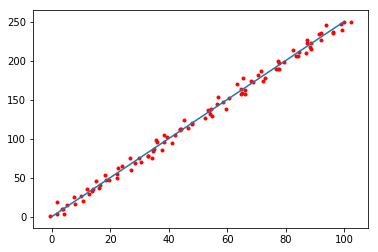### 计算图

Computational Graph 指的是一系列的操作，包括输出的数据。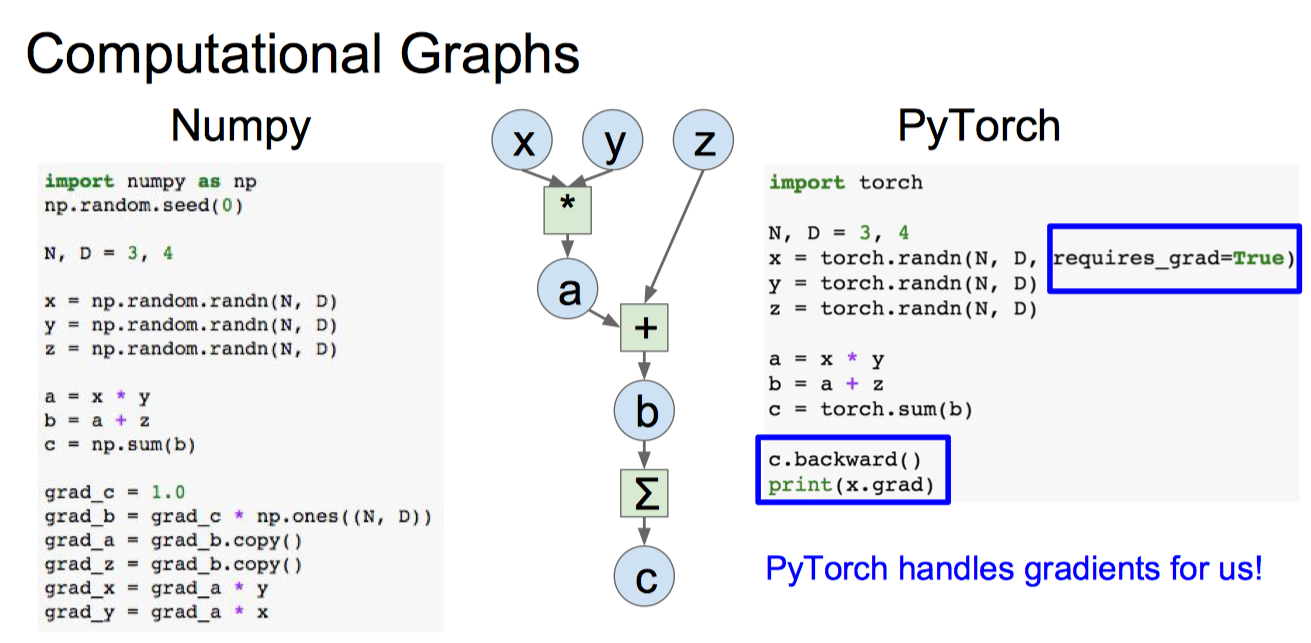TensorFlow 的数据流图的例子，数据经过节点被处理，然后输出，就像水一样流动: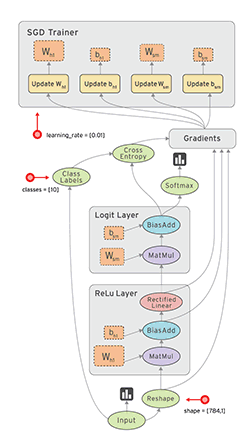Pytorch 是动态图，每一次训练，都会销毁图并重新创建，这样做花销很大，但是更加灵活。 而 Tensorflow 是静态图，一旦定义训练时就不能修改。Pytorch 合并 caffe2 发布 1.0 版本之后引入静态图，而 Tensorflow 已经发布 Eager Execution 引入动态图。但相对来说还是 Pytorch 更加灵活。

Pytorch 会自动跟踪计算图的操作，在计算图执行完成后，调用 backward 计算梯度。很久以前数据需要使用 Variable 封装，像这样的:import torch
x = torch.randn(5, 5)
y = torch.randn(5, 5)
z = x + y

False

x = torch.randn(5, 5, requires_grad=True)
y = torch.randn(5, 5)
z = x + y

True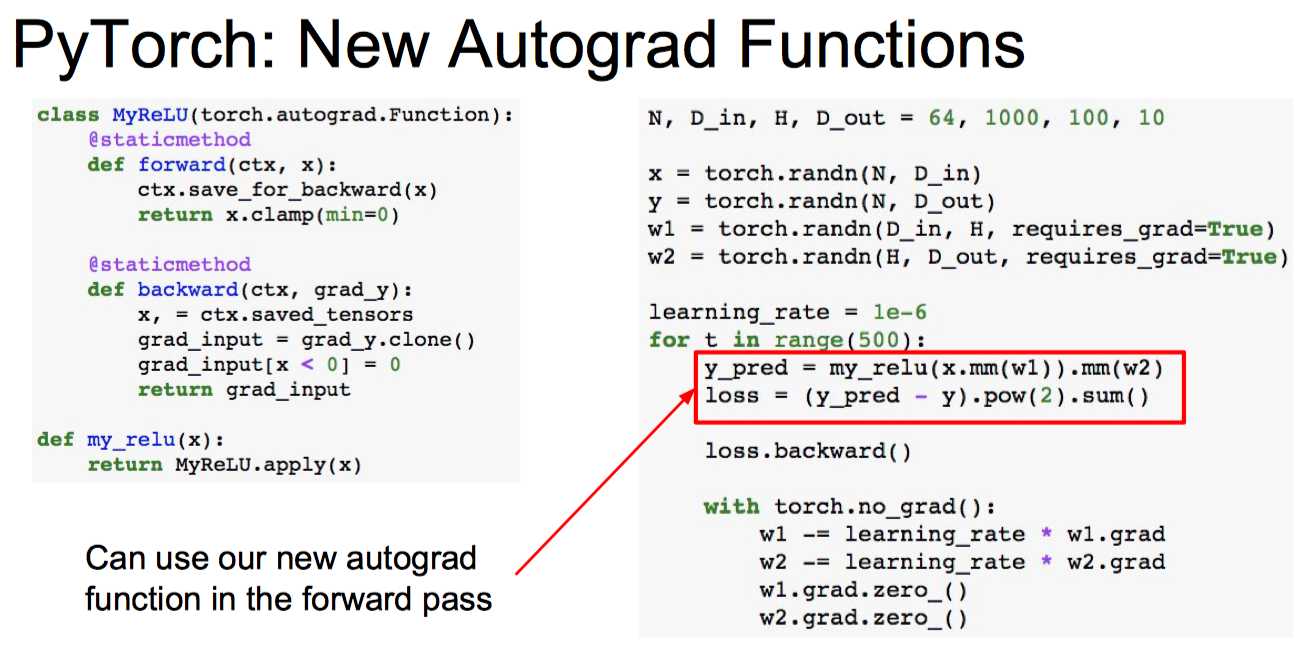backward 之后更新完参数需要清除梯度，torch.no_grad 是指在此的计算不创建图的节点。

### 实现线性拟合

$$d = \left| \frac { A x _ { 0 } + B y _ { 0 } + C } { \sqrt { A ^ { 2 } + B ^ { 2 } } } \right|$$

$$d = \left| \frac { a x _ { 0 } - y _ { 0 } + b } { \sqrt { a ^ { 2 } + 1 } } \right|$$

$$L = \frac{1}{2N}\sum_i \frac{(a x _ { i } - y _ { i } + b)^2}{a^2+1}$$

import numpy as np
import random
import matplotlib.pyplot as plt
# 在0-2*pi的区间上生成100个点作为输入数据
X = np.linspace(0, 100, 100,endpoint=True)
a, b = 2.5, 1.0
Y = a*X+b

# 对输入数据加入gauss噪声
# 定义gauss噪声的均值和方差
mu = 0
sigma = 2
Nx, Ny = X.copy(), Y.copy()
for i in range(X.shape):
Nx[i] += random.gauss(mu,sigma)
Ny[i] += random.gauss(mu,sigma)

# 画出这些点
plt.plot(X, Y)
plt.scatter(Nx, Ny, marker='.', color='r')
plt.show()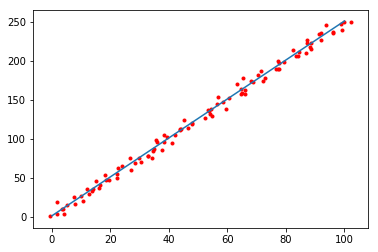X = torch.from_numpy(Nx)
Y = torch.from_numpy(Ny)
X = X.float()
Y = Y.float()
lr = 1e-5
iA, iB = torch.Tensor(), torch.Tensor()
# 需要计算梯度

# 记录最好的结果
best_loss = float("inf")
best_a = 0.0
best_b = 0.0
loss_list = []
max_epochs = 1000

# 梯度下降
for _ in range(max_epochs):
# 计算 loss
loss = torch.mean((iA*X-Y+iB)**2/(iA**2+1))
# 反向传播
loss.backward()
# 更新参数
cur_loss = loss.item()
if cur_loss<best_loss:
best_a = iA.item()
best_b = iB.item()
best_loss = cur_loss
loss_list.append(cur_loss)

# loss 曲线
plt.plot(list(range(max_epochs)), loss_list)
plt.show()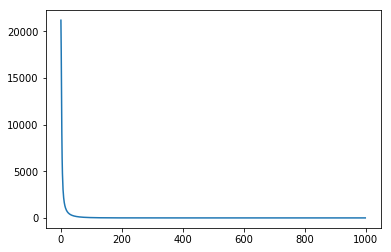best_a, best_b, best_loss

(2.505525827407837, 0.027117641642689705, 4.3863372802734375)

nX = np.linspace(0, 100, 100,endpoint=True)
plt.plot(nX, best_a*nX+best_b)
plt.scatter(Nx, Ny, marker='.', color='r')
plt.show()